# Localization in a commutative algebra

(diff) ← Older revision | Latest revision (diff) | Newer revision → (diff)

A transition from a commutative ringto the ring of fractions (cf. Fractions, ring of)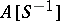, whereis a subset of. The ringcan be defined as the solution of the problem of a universal mapping frominto a ring under which all elements ofbecome invertible. However, there are explicit constructions for:

1) as the set of fractions of the form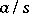, where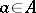andis a product of elements of(two fractionsandare regarded as equivalent if and only if there is an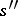that is a product of elements ofand is such that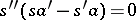; fractions are added and multiplied by the usual rules);

2) as the quotient ring of the ring of polynomials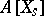,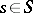, with respect to the ideal generated by the polynomials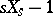,;

3) as the inductive limit of an inductive system of-modules, whereruns through a naturally-ordered free commutative monoid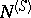. All the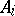are isomorphic to, and the homomorphisms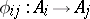with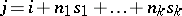coincide with multiplication by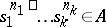.

The ringis canonically mapped intoand converts the latter into an-algebra. This mappingis injective if and only ifdoes not contain any divisor of zero in. On the other hand, ifcontains a nilpotent element, then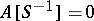.

Without loss of generality the setcan be assumed to be closed with respect to products (such a set is known as multiplicative, or as a multiplicative system). In this case the ringis also denoted byor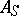. The most important examples of multiplicative systems are the following:

a) the set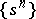of all powers of an element of;

b) the set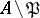, that is, the complement of a prime ideal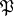. The corresponding ring of fractions is local and is denoted by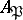;

c) the setof all non-divisors of zero in.

The ring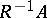is called the complete ring of fractions of. Ifis integral, then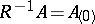is a field of fractions.

The operation of localization carries over with no difficulty to arbitrary-modulesif one setsThe transition fromto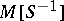is an exact functor. In other words, the-moduleis flat. Localization commutes with direct sums and inductive limits.

From the geometrical point of view localization means transition to an open subset. More precisely, forthe spectrum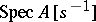is canonically identified with the open (in the Zariski topology) subset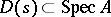consisting of the prime idealsnot containing. Moreover, this operation makes it possible to associate with each-modulea quasi-coherent sheaf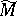on the affine scheme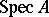for which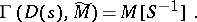Localization can be regarded as an operation that makes it possible to invert morphisms of multiplication by anin the category of-modules. In this approach the operation of localization admits a wide generalization to arbitrary categories (see Localization in categories).

How to Cite This Entry:
Localization in a commutative algebra. Encyclopedia of Mathematics. URL: http://encyclopediaofmath.org/index.php?title=Localization_in_a_commutative_algebra&oldid=18943
This article was adapted from an original article by V.I. Danilov (originator), which appeared in Encyclopedia of Mathematics - ISBN 1402006098. See original article